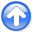Regularization is model stylingNext: WELLS NOT MATCHING THE Up: Missing-data program Previous: Matrix approach to missing

### Operator approach to missing data

For the operator approach to the fitting goal, we rewrite it aswhere(3)

Notice the introduction of the new diagonal matrix, called a masking matrix or a constraint-mask matrix because it multiplies constrained variables by zero leaving freely adjustable variables untouched. Experience shows that a better name than mask matrix'' is selector matrix'' because what comes out of it, that which is selected, is a less-confusing name for it than which is rejected. With a selector matrix, the whole data space seems freely adjustable, both the missing data values and known values. We see that the CD method does not change the known (constrained) values. In general, we derive the fitting goal (3) by:(4)(5)(6)(7)(8)

As usual, we find a direction to goby the gradient of the residual energy.(9)

We begin the calculation with the known data values where missing data values are replaced by zeros, namely. Filter this data, getting, and load it into the residual. With this initialization completed, we begin an iteration loop. First we computefrom equation (9).(10)applies a crosscorrelation of the filter to the residual and thensets to zero any changes proposed to known data values. Next, compute the change in residualfrom the proposed change in the data.(11)

Equation (11) applies the filtering again. Then, use the method of steepest descent (or conjugate direction) to choose the appropriate scaling (or inclusion of previous step) ofand, and updateand, accordingly, and iterate.

The subroutine to find missing data is mis1(). It assumes that zero values in the input data correspond to missing data locations. It uses our convolution operator tcai1(). You can also check the book Index for other operators and modules.

user/gee/mis1.c
 void mis1(int niter /* number of iterations */, float *xx /* data/model */, const bool *known /* mask for known data */, const char *step /* solver */) /*< interpolate >*/ { switch (step) { case 'g': /* conjugate gradients */ sf_solver (tcai1_lop, sf_cgstep, nx, ny, xx, zero, niter, "x0", xx, "known", known, "end"); sf_cgstep_close(); break; case 'd': /* conjugate directions */ sf_cdstep_init(); sf_solver (tcai1_lop, sf_cdstep, nx, ny, xx, zero, niter, "x0", xx, "known", known, "end"); sf_cdstep_close(); break; default: sf_error("%s: unknown step %s",__FILE__,step); break; } } 

I sought reference material on conjugate gradients with constraints and did not find anything, leaving me to fear that this chapter was in error, and I had lost the magic property of convergence in a finite number of iterations. I tested the code, and it did converge in a finite number of iterations. The explanation is that these constraints are almost trivial. We pretended we had extra variables, and computed afor each. Usingwe then set the gradientto zero, therefore making no changes to anything, like as if we had never calculated the extras.Regularization is model stylingNext: WELLS NOT MATCHING THE Up: Missing-data program Previous: Matrix approach to missing

2014-12-03